# Difference: LPRelaxation (1 vs. 4)

Line: 1 to 1

 META TOPICPARENT name="IntegerProgramming"

# Linear Programming Relaxation

Line: 28 to 28
-- MichaelOSullivan - 23 Apr 2008
Changed:
<
<
 META FILEATTACHMENT attachment="latexe4b8d6a489763d604b98ebb232748397.png" attr="h" comment="" date="1208928939" name="latexe4b8d6a489763d604b98ebb232748397.png" stream="GLOB(0x91ce7a8)" tmpFilename="latexe4b8d6a489763d604b98ebb232748397.png" user="MichaelOSullivan" version="1" attachment="latexa8e4b5589e54347e2ba4810c294f4b5c.png" attr="h" comment="" date="1208928939" name="latexa8e4b5589e54347e2ba4810c294f4b5c.png" stream="GLOB(0x91ce6e8)" tmpFilename="latexa8e4b5589e54347e2ba4810c294f4b5c.png" user="MichaelOSullivan" version="1" attachment="latexed6a2ff354d3aae18c22e0d46c66cf70.png" attr="h" comment="" date="1208928939" name="latexed6a2ff354d3aae18c22e0d46c66cf70.png" stream="GLOB(0x91ce6b8)" tmpFilename="latexed6a2ff354d3aae18c22e0d46c66cf70.png" user="MichaelOSullivan" version="1" attachment="latex8d0eb5d826b9c06c5fee8895f5b0170c.png" attr="h" comment="" date="1208928939" name="latex8d0eb5d826b9c06c5fee8895f5b0170c.png" stream="GLOB(0x91cebd4)" tmpFilename="latex8d0eb5d826b9c06c5fee8895f5b0170c.png" user="MichaelOSullivan" version="1" attachment="latex31c05bb52a9dc2026e0ec5066218ee90.png" attr="h" comment="" date="1208928939" name="latex31c05bb52a9dc2026e0ec5066218ee90.png" stream="GLOB(0x91cef40)" tmpFilename="latex31c05bb52a9dc2026e0ec5066218ee90.png" user="MichaelOSullivan" version="1" attachment="latexd33e16fed8e048f80ced5c47e80f0887.png" attr="h" comment="" date="1208928939" name="latexd33e16fed8e048f80ced5c47e80f0887.png" stream="GLOB(0x91cf000)" tmpFilename="latexd33e16fed8e048f80ced5c47e80f0887.png" user="MichaelOSullivan" version="1" attachment="latexb3f085e80a23f892fcf4206fb696265f.png" attr="h" comment="" date="1208928939" name="latexb3f085e80a23f892fcf4206fb696265f.png" stream="GLOB(0x91ceb68)" tmpFilename="latexb3f085e80a23f892fcf4206fb696265f.png" user="MichaelOSullivan" version="1"
>
>
 META FILEATTACHMENT attachment="latexfe0d9bfd0a8ef8ec492ce8c60d14a2ef.png" attr="h" comment="" date="1542935666" name="latexfe0d9bfd0a8ef8ec492ce8c60d14a2ef.png" user="BaseUserMapping_333" version="1" attachment="latex765f07137e5ccaaedb71410ea9e425bb.png" attr="h" comment="" date="1542935666" name="latex765f07137e5ccaaedb71410ea9e425bb.png" user="BaseUserMapping_333" version="1" attachment="latex5d2941f50300797f70755f99be3de882.png" attr="h" comment="" date="1542935666" name="latex5d2941f50300797f70755f99be3de882.png" user="BaseUserMapping_333" version="1" attachment="latex851a1c8af71a8e391bb2346732994bcf.png" attr="h" comment="" date="1542935667" name="latex851a1c8af71a8e391bb2346732994bcf.png" user="BaseUserMapping_333" version="1" attachment="latex241d5906b695abb053ee06c80cb3f153.png" attr="h" comment="" date="1542935667" name="latex241d5906b695abb053ee06c80cb3f153.png" user="BaseUserMapping_333" version="1" attachment="latex51ac9d0902422300dbc49d0461cce956.png" attr="h" comment="" date="1542935667" name="latex51ac9d0902422300dbc49d0461cce956.png" user="BaseUserMapping_333" version="1" attachment="latex07dca684d2fca4a81efb6df6c6e2697f.png" attr="h" comment="" date="1542935667" name="latex07dca684d2fca4a81efb6df6c6e2697f.png" user="BaseUserMapping_333" version="1"

#### Revision 32008-04-23 - MichaelOSullivan

Line: 1 to 1

 META TOPICPARENT name="IntegerProgramming"

# Linear Programming Relaxation

Line: 13 to 13

• Most network flow problems are also naturally integer;
• Some scheduling problems are naturally integer.
Changed:
<
<
In some mathematical programming software, the integer restrictions may be easily realxed and the software will solve the LP relaxation instead. For example, in AMPL the LP relaxation may be solved simply by turning on the relax_integrality option:
>
>

## Using AMPL

In AMPL the LP relaxation may be solved simply by turning on the relax_integrality option:

option relax_integrality 1;

Line: 21 to 24

option relax_integrality 0;

Changed:
<
<
means that the integer programme will be solved again.
>
>
-- MichaelOSullivan - 23 Apr 2008

#### Revision 22008-04-23 - MichaelOSullivan

Line: 1 to 1

 META TOPICPARENT name="IntegerProgramming"
Changed:
<
<

>
>

# Linear Programming Relaxation

Changed:
<
<
The Linear Programming (LP) relaxation is the same as the integer programme, except we "relax" the integer variables to allow them to take fractional values. The integer programme's feasible region lies within the feasible region of the LP relaxation (at points where the integer variables have integer values). Therefore the integer restrictions cause the optimal objective function value to be worse in the integer programme as compared to the LP relaxation. However, if a solution $x$ of the LP relaxation has integer values for the integer variables, then $x$ also solves the integer programme. In some cases, if the solution values for the integer variables are large, then rounding the LP relaxation solution may give a good solution to the integer programme. However, you need to make sure that the rounded solution is not infeasible!

For some classes of problem the LP relaxation gives naturally integer solutions:

• An $m \times m$ matrix $M$ is unimodular if and only if its determinant $|M|$ is equal to 1 or –1;
• An $m \times n$ matrix $M$ is totally unimodular if and only if every $m \times m$ non-singular submatrix of $M$ is unimodular;
• If the constraint matrix $A$ and the right-hand side vector $b$ of a mixed-integer programme are totally unimodular and integer respectively, then the mixed-integer programme is naturally integer and the LP relaxation solution is the optimal solution

• The transportation problem is an example of one such problem;
• Most network flow problems are also naturally integer;
• Some scheduling problems are naturally integer.

In AMPL, the LP relaxation may be solved simply by turning on the {\tt relax_integrality} option:

\begin{verbatim}
option relax_integrality 1;
\end{verbatim}

To turn {\tt relax_integrality} off, simply set it to 0.

>
>
The Linear Programming (LP) relaxation is the same as the original integer programme, except we "relax" the integer variables to allow them to take fractional values. The integer programme's feasible region lies within the feasible region of the LP relaxation (at points where the integer variables have integer values). Therefore the integer restrictions cause the optimal objective function value to be worse in the integer programme as compared to the LP relaxation. However, if a solution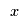of the LP relaxation has integer values for the integer variables, thenalso solves the integer programme. In some cases, if the solution values for the integer variables are large, then rounding the LP relaxation solution may give a good solution to the integer programme. However, you need to make sure that the rounded solution is not infeasible!

For some classes of problem the LP relaxation gives naturally integer solutions:

• An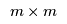matrix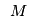is unimodular if and only if its determinant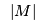is equal to 1 or –1;
• An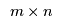matrixis totally unimodular if and only if everynon-singular submatrix ofis unimodular;
• If the constraint matrix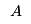and the right-hand side vector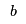of a mixed-integer programme are totally unimodular and integer respectively, then the mixed-integer programme is naturally integer and the LP relaxation solution is the optimal solution *The transportation problem is an example of one such problem;
• Most network flow problems are also naturally integer;
• Some scheduling problems are naturally integer.

In some mathematical programming software, the integer restrictions may be easily realxed and the software will solve the LP relaxation instead. For example, in AMPL the LP relaxation may be solved simply by turning on the relax_integrality option:

option relax_integrality 1;

Turning it off:
option relax_integrality 0;

means that the integer programme will be solved again.
-- MichaelOSullivan - 23 Apr 2008 \ No newline at end of file
>
>
 META FILEATTACHMENT attachment="latexe4b8d6a489763d604b98ebb232748397.png" attr="h" comment="" date="1208928939" name="latexe4b8d6a489763d604b98ebb232748397.png" stream="GLOB(0x91ce7a8)" tmpFilename="latexe4b8d6a489763d604b98ebb232748397.png" user="MichaelOSullivan" version="1" attachment="latexa8e4b5589e54347e2ba4810c294f4b5c.png" attr="h" comment="" date="1208928939" name="latexa8e4b5589e54347e2ba4810c294f4b5c.png" stream="GLOB(0x91ce6e8)" tmpFilename="latexa8e4b5589e54347e2ba4810c294f4b5c.png" user="MichaelOSullivan" version="1" attachment="latexed6a2ff354d3aae18c22e0d46c66cf70.png" attr="h" comment="" date="1208928939" name="latexed6a2ff354d3aae18c22e0d46c66cf70.png" stream="GLOB(0x91ce6b8)" tmpFilename="latexed6a2ff354d3aae18c22e0d46c66cf70.png" user="MichaelOSullivan" version="1" attachment="latex8d0eb5d826b9c06c5fee8895f5b0170c.png" attr="h" comment="" date="1208928939" name="latex8d0eb5d826b9c06c5fee8895f5b0170c.png" stream="GLOB(0x91cebd4)" tmpFilename="latex8d0eb5d826b9c06c5fee8895f5b0170c.png" user="MichaelOSullivan" version="1" attachment="latex31c05bb52a9dc2026e0ec5066218ee90.png" attr="h" comment="" date="1208928939" name="latex31c05bb52a9dc2026e0ec5066218ee90.png" stream="GLOB(0x91cef40)" tmpFilename="latex31c05bb52a9dc2026e0ec5066218ee90.png" user="MichaelOSullivan" version="1" attachment="latexd33e16fed8e048f80ced5c47e80f0887.png" attr="h" comment="" date="1208928939" name="latexd33e16fed8e048f80ced5c47e80f0887.png" stream="GLOB(0x91cf000)" tmpFilename="latexd33e16fed8e048f80ced5c47e80f0887.png" user="MichaelOSullivan" version="1" attachment="latexb3f085e80a23f892fcf4206fb696265f.png" attr="h" comment="" date="1208928939" name="latexb3f085e80a23f892fcf4206fb696265f.png" stream="GLOB(0x91ceb68)" tmpFilename="latexb3f085e80a23f892fcf4206fb696265f.png" user="MichaelOSullivan" version="1"

#### Revision 12008-04-23 - MichaelOSullivan

Line: 1 to 1
>
>
 META TOPICPARENT name="IntegerProgramming"

# L(inear) P(rogramming) Relaxation

The Linear Programming (LP) relaxation is the same as the integer programme, except we "relax" the integer variables to allow them to take fractional values. The integer programme's feasible region lies within the feasible region of the LP relaxation (at points where the integer variables have integer values). Therefore the integer restrictions cause the optimal objective function value to be worse in the integer programme as compared to the LP relaxation. However, if a solution $x$ of the LP relaxation has integer values for the integer variables, then $x$ also solves the integer programme. In some cases, if the solution values for the integer variables are large, then rounding the LP relaxation solution may give a good solution to the integer programme. However, you need to make sure that the rounded solution is not infeasible!

For some classes of problem the LP relaxation gives naturally integer solutions:

• An $m \times m$ matrix $M$ is unimodular if and only if its determinant $|M|$ is equal to 1 or –1;
• An $m \times n$ matrix $M$ is totally unimodular if and only if every $m \times m$ non-singular submatrix of $M$ is unimodular;
• If the constraint matrix $A$ and the right-hand side vector $b$ of a mixed-integer programme are totally unimodular and integer respectively, then the mixed-integer programme is naturally integer and the LP relaxation solution is the optimal solution

• The transportation problem is an example of one such problem;
• Most network flow problems are also naturally integer;
• Some scheduling problems are naturally integer.

In AMPL, the LP relaxation may be solved simply by turning on the {\tt relax_integrality} option:

\begin{verbatim}
option relax_integrality 1;
\end{verbatim}

To turn {\tt relax_integrality} off, simply set it to 0.

-- MichaelOSullivan - 23 Apr 2008

Copyright © 2008-2023 by the contributing authors. All material on this collaboration platform is the property of the contributing authors.
Ideas, requests, problems regarding TWiki? Send feedback